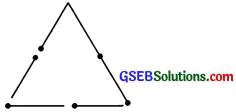# GSEB Solutions Class 6 Maths Chapter 5 Understanding Elementary Shapes Ex 5.6

Gujarat Board GSEB Textbook Solutions Class 6 Maths Chapter 5 Understanding Elementary Shapes Ex 5.6 Textbook Questions and Answers.

## Gujarat Board Textbook Solutions Class 6 Maths Chapter 5 Understanding Elementary Shapes Ex 5.6

Question 1.
Name the type of following triangles:
(a) Triangle with lengths of sides 7 cm, 8 cm and 9 cm.
(b) ∆ABC with AB = 8.7 cm, AC = 7 cm and BC = 6 cm.
(c) ∆PQR such that PQ = QR = PR = 5 cm.
(d) ∆DEF with m$$\angle D$$ 90°
(e) ∆XYZ with m$$\angle Y$$ = 90° and XY = YZ.
(f) ∆LMN with m$$\angle L$$ = 30°, m$$\angle M$$ = 70° and m$$\angle N$$ = 80°.
Solution:
(a) Since, 7 cm ≠ 8 cm ≠ 9 cm
i.e. all the sides are unequal The triangle is a scalene triangle.

(b) Since, AB ≠ BC ≠ AC
i.e. all the sides are unequal.
∆ABC is a scalene triangle.

(c) Since, PQ ≠ QR ≠ PR
i.e. all the sides are equal
∆PQR is an equilateral triangle.

(d) Since m$$\angle D$$ = 90°
∆DEF is a right angled triangle.

(e) Since, m$$\angle Y$$ = 90° and XY = YZ
i.e. two sides of the triangle are equal.
∆XYZ is an isosceles right triangle.

(f) Since m$$\angle L$$ = 30°, m$$\angle M$$ = 70°, m$$\angle N$$ = 80°
i.e. all the angles of ∆LMN are acute.
∆LMN is an acute angled triangle.Question 2.
Mach the following: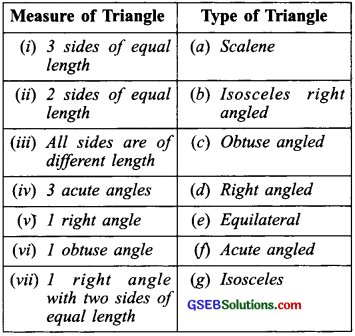Solution: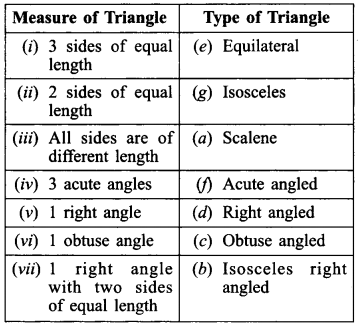Question 3.
Name each of the following triangles in two different ways: (you may judge the nature of the angle by observation)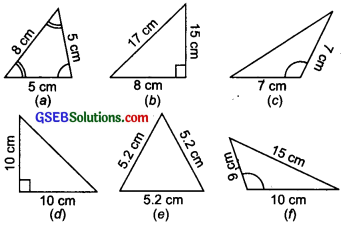Solution: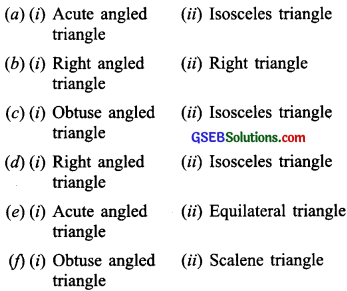Question 4.
Try to construct triangles using matchsticks. Some are shown here. Can you make a triangle with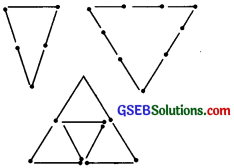(a) 3 matchsticks?
(b) 4 matchsticks?
(c) 5 matchsticks?
(d) 6 matchsticks?
(Remember you have to use all the available matchsticks in each case)
Name the type of triangle in each case. If you cannot make a triangle, think of reasons for it.
Solution:
(a) Yes, we can make an equilateral triangle with 3 matchsticks as shown in the figure.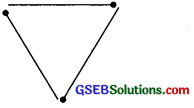(b) No, we cannot make a triangle, using 4 matchsticks. [ The sum of any two sides must be greater than the third, which is not possible here.]

(c) Yes, an isosceles triangle can be made using 5 matchsticks, as shown in the figure.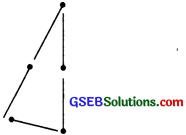(d) Yes, an equilateral triangle can be made using 6 matchsticks as shown in the figure: